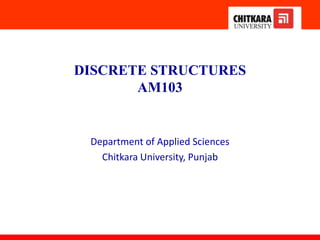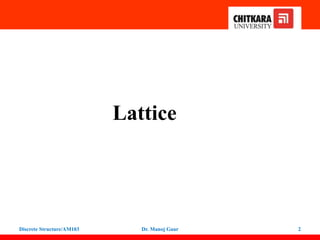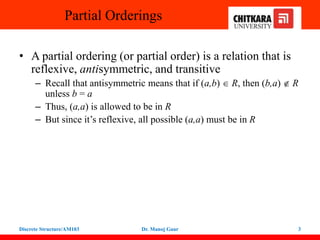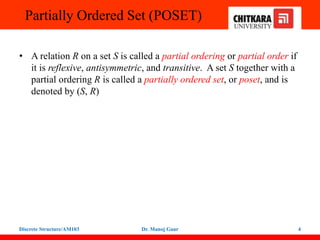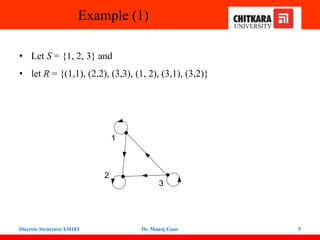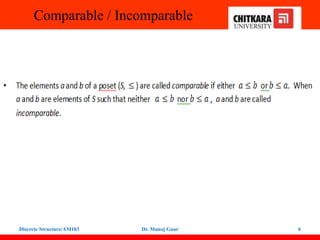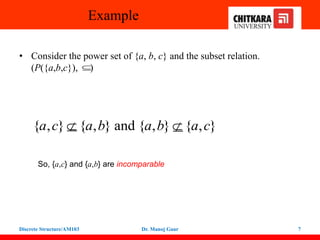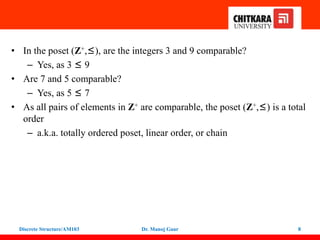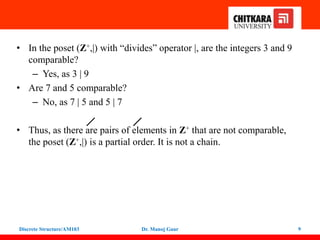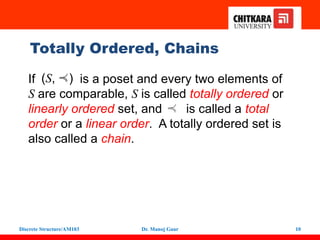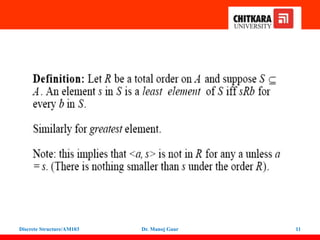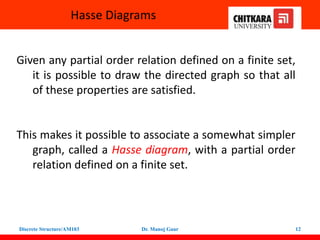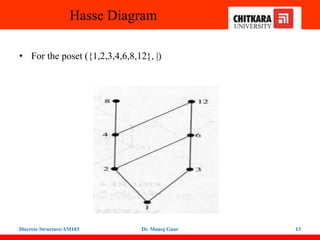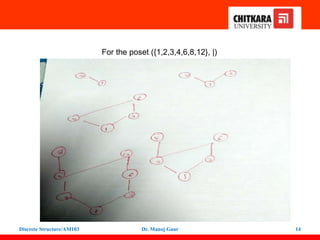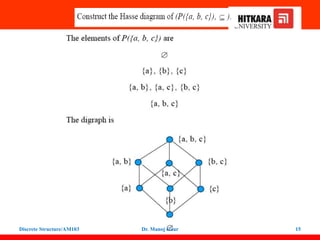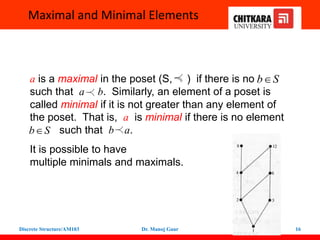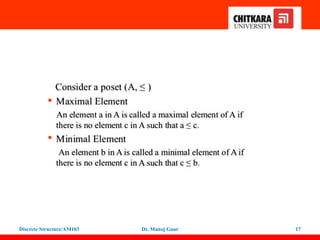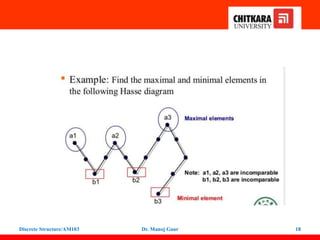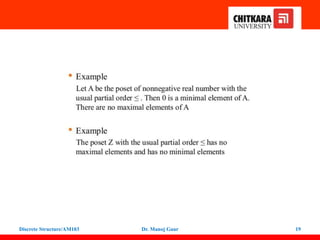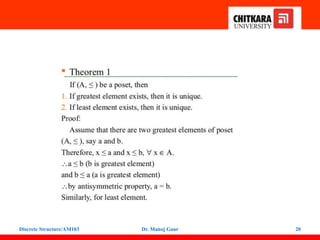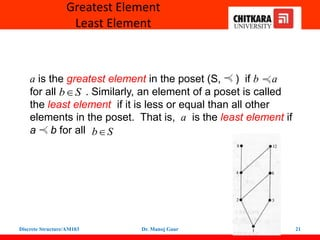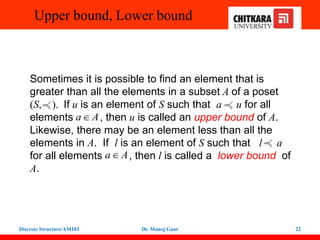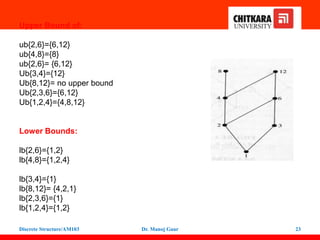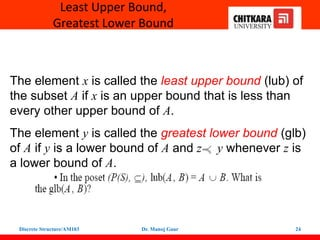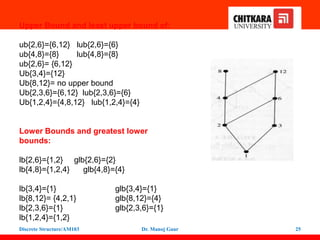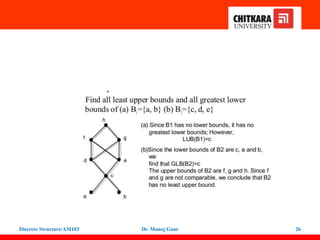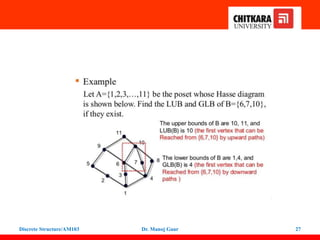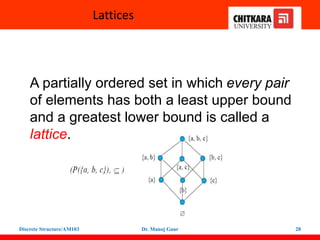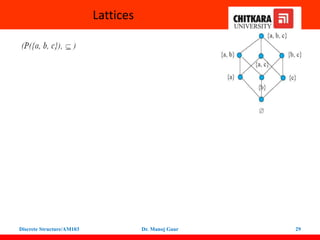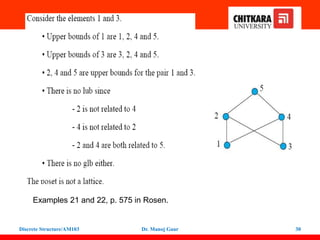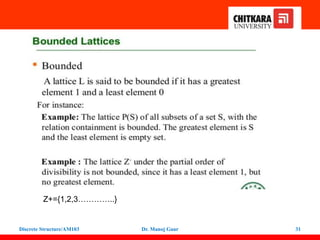1 de 31

### AM103(Unit3).pptx

1. DISCRETE STRUCTURES AM103 Department of Applied Sciences Chitkara University, Punjab
2. Lattice 2 Discrete Structure/AM103 Dr. Manoj Gaur
3. Partial Orderings • A partial ordering (or partial order) is a relation that is reflexive, antisymmetric, and transitive – Recall that antisymmetric means that if (a,b)  R, then (b,a) R unless b = a – Thus, (a,a) is allowed to be in R – But since it’s reflexive, all possible (a,a) must be in R 3 Discrete Structure/AM103 Dr. Manoj Gaur
4. Partially Ordered Set (POSET) • A relation R on a set S is called a partial ordering or partial order if it is reflexive, antisymmetric, and transitive. A set S together with a partial ordering R is called a partially ordered set, or poset, and is denoted by (S, R) 4 Discrete Structure/AM103 Dr. Manoj Gaur
5. Example (1) • Let S = {1, 2, 3} and • let R = {(1,1), (2,2), (3,3), (1, 2), (3,1), (3,2)} 5 1 2 3 Discrete Structure/AM103 Dr. Manoj Gaur
6. Comparable / Incomparable 6 Discrete Structure/AM103 Dr. Manoj Gaur
7. Example • Consider the power set of {a, b, c} and the subset relation. (P({a,b,c}), ) 7 { , } { , } and { , } { , } a c a b a b a c   So, {a,c} and {a,b} are incomparable  Discrete Structure/AM103 Dr. Manoj Gaur
8. 8 • In the poset (Z+,≤), are the integers 3 and 9 comparable? – Yes, as 3 ≤ 9 • Are 7 and 5 comparable? – Yes, as 5 ≤ 7 • As all pairs of elements in Z+ are comparable, the poset (Z+,≤) is a total order – a.k.a. totally ordered poset, linear order, or chain Discrete Structure/AM103 Dr. Manoj Gaur
9. 9 • In the poset (Z+,|) with “divides” operator |, are the integers 3 and 9 comparable? – Yes, as 3 | 9 • Are 7 and 5 comparable? – No, as 7 | 5 and 5 | 7 • Thus, as there are pairs of elements in Z+ that are not comparable, the poset (Z+,|) is a partial order. It is not a chain. Discrete Structure/AM103 Dr. Manoj Gaur
10. 10 (S, ) If is a poset and every two elements of S are comparable, S is called totally ordered or linearly ordered set, and is called a total order or a linear order. A totally ordered set is also called a chain. Totally Ordered, Chains Discrete Structure/AM103 Dr. Manoj Gaur
11. 11 Discrete Structure/AM103 Dr. Manoj Gaur
12. 12 Hasse Diagrams Given any partial order relation defined on a finite set, it is possible to draw the directed graph so that all of these properties are satisfied. This makes it possible to associate a somewhat simpler graph, called a Hasse diagram, with a partial order relation defined on a finite set. Discrete Structure/AM103 Dr. Manoj Gaur
13. 13 Hasse Diagram • For the poset ({1,2,3,4,6,8,12}, |) Discrete Structure/AM103 Dr. Manoj Gaur
14. 14 For the poset ({1,2,3,4,6,8,12}, |) Discrete Structure/AM103 Dr. Manoj Gaur
15. 15 Discrete Structure/AM103 Dr. Manoj Gaur
16. 16 a is a maximal in the poset (S, ) if there is no such that a b. Similarly, an element of a poset is called minimal if it is not greater than any element of the poset. That is, a is minimal if there is no element such that b a. It is possible to have multiple minimals and maximals. Maximal and Minimal Elements b S  b S  Discrete Structure/AM103 Dr. Manoj Gaur
17. 17 Discrete Structure/AM103 Dr. Manoj Gaur
18. 18 Discrete Structure/AM103 Dr. Manoj Gaur
19. 19 Discrete Structure/AM103 Dr. Manoj Gaur
20. 20 Discrete Structure/AM103 Dr. Manoj Gaur
21. 21 a is the greatest element in the poset (S, ) if b a for all . Similarly, an element of a poset is called the least element if it is less or equal than all other elements in the poset. That is, a is the least element if a b for all Greatest Element Least Element b S  b S  Discrete Structure/AM103 Dr. Manoj Gaur
22. 22 Upper bound, Lower bound Sometimes it is possible to find an element that is greater than all the elements in a subset A of a poset (S, ). If u is an element of S such that a u for all elements , then u is called an upper bound of A. Likewise, there may be an element less than all the elements in A. If l is an element of S such that l a for all elements , then l is called a lower bound of A. a A  a A  Discrete Structure/AM103 Dr. Manoj Gaur
23. 23 Upper Bound of: ub{2,6}={6,12} ub{4,8}={8} ub{2,6}= {6,12} Ub{3,4}={12} Ub{8,12}= no upper bound Ub{2,3,6}={6,12} Ub{1,2,4}={4,8,12} Lower Bounds: lb{2,6}={1,2} lb{4,8}={1,2,4} lb{3,4}={1} lb{8,12}= {4,2,1} lb{2,3,6}={1} lb{1,2,4}={1,2} Discrete Structure/AM103 Dr. Manoj Gaur
24. 24 Least Upper Bound, Greatest Lower Bound The element x is called the least upper bound (lub) of the subset A if x is an upper bound that is less than every other upper bound of A. The element y is called the greatest lower bound (glb) of A if y is a lower bound of A and z y whenever z is a lower bound of A. Discrete Structure/AM103 Dr. Manoj Gaur
25. 25 Upper Bound and least upper bound of: ub{2,6}={6,12} lub{2,6}={6} ub{4,8}={8} lub{4,8}={8} ub{2,6}= {6,12} Ub{3,4}={12} Ub{8,12}= no upper bound Ub{2,3,6}={6,12} lub{2,3,6}={6} Ub{1,2,4}={4,8,12} lub{1,2,4}={4} Lower Bounds and greatest lower bounds: lb{2,6}={1,2} glb{2,6}={2} lb{4,8}={1,2,4} glb{4,8}={4} lb{3,4}={1} glb{3,4}={1} lb{8,12}= {4,2,1} glb{8,12}={4} lb{2,3,6}={1} glb{2,3,6}={1} lb{1,2,4}={1,2} Discrete Structure/AM103 Dr. Manoj Gaur
26. 26 Discrete Structure/AM103 Dr. Manoj Gaur
27. 27 Discrete Structure/AM103 Dr. Manoj Gaur
28. 28 Lattices A partially ordered set in which every pair of elements has both a least upper bound and a greatest lower bound is called a lattice. Discrete Structure/AM103 Dr. Manoj Gaur
29. 29 Lattices Discrete Structure/AM103 Dr. Manoj Gaur
30. 30 Examples 21 and 22, p. 575 in Rosen. Discrete Structure/AM103 Dr. Manoj Gaur
31. 31 Z+={1,2,3…………..} Discrete Structure/AM103 Dr. Manoj Gaur